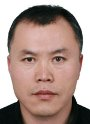Electron. J. Differential Equations, Vol. 2018 (2018), No. 131, pp. 1-13.

### Optimal distributed control problem for the modified Swift-Hohenberg equations Bing Sun

Abstract:
This article concerns the optimal distributed control for the modified Swift-Hohenberg equation. Using the Dubovitskii and Milyutin functional analytical approach, we prove the Pontryagin maximum principle of the controlled modified Swift-Hohenberg equation. A necessary optimality condition is established for the problem in fixed final time horizon case. Also we indicate how to utilize the obtained results.

Submitted May 21, 2018. Published June 27, 2018.
Math Subject Classifications: 35Q35, 49B22, 49K20.
Key Words: Maximum principle; optimal distributed control; necessary optimality condition; Swift-Hohenberg equation.

Show me the PDF file (237 KB), TEX file for this article.Bing Sun School of Mathematics and Statistics Beijing Institute of Technology Beijing 100081, China email: sunamss@gmail.com